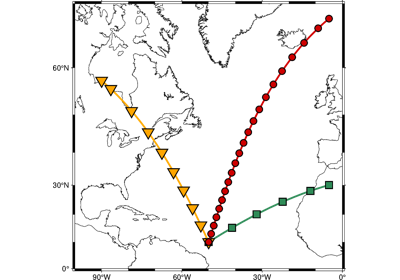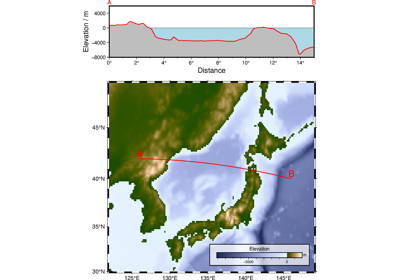# pygmt.project

pygmt.project(data=None, x=None, y=None, z=None, outfile=None, *, azimuth=None, center=None, endpoint=None, convention=None, generate=None, length=None, flat_earth=None, unit=None, sort=None, pole=None, verbose=None, width=None, ellipse=None, coltypes=None, **kwargs)[source]

Project data onto lines or great circles, or generate tracks.

Project reads arbitrary $$(x, y [, z])$$ data and returns any combination of $$(x, y, z, p, q, r, s)$$, where $$(p, q)$$ are the coordinates in the projection, $$(r, s)$$ is the position in the $$(x, y)$$ coordinate system of the point on the profile ($$q = 0$$ path) closest to $$(x, y)$$, and $$z$$ is all remaining columns in the input (beyond the required $$x$$ and $$y$$ columns).

Alternatively, project may be used to generate $$(r, s, p)$$ triplets at equal increments along a profile using the generate parameter. In this case, the value of data is ignored (you can use, e.g., data=None).

Projections are defined in any (but only) one of three ways:

1. By a center and an azimuth in degrees clockwise from North.

2. By a center and endpoint of the projection path.

3. By a center and a pole position.

To spherically project data along a great circle path, an oblique coordinate system is created which has its equator along that path, and the zero meridian through the Center. Then the oblique longitude ($$p$$) corresponds to the distance from the Center along the great circle, and the oblique latitude ($$q$$) corresponds to the distance perpendicular to the great circle path. When moving in the increasing ($$p$$) direction, (toward B or in the azimuth direction), the positive ($$q$$) direction is to your left. If a Pole has been specified, then the positive ($$q$$) direction is toward the pole.

To specify an oblique projection, use the pole parameter to set the pole. Then the equator of the projection is already determined and the center parameter is used to locate the $$p = 0$$ meridian. The center cx/cy will be taken as a point through which the $$p = 0$$ meridian passes. If you do not care to choose a particular point, use the South pole (cx = 0, cy = -90).

Data can be selectively windowed by using the length and width parameters. If width is used, the projection width is set to use only data with $$w_{min} < q < w_{max}$$. If length is set, then the length is set to use only those data with $$l_{min} < p < l_{max}$$. If the endpoint parameter has been used to define the projection, then length="w" may be used to window the length of the projection to exactly the span from O to B.

Flat Earth (Cartesian) coordinate transformations can also be made. Set flat_earth=True and remember that azimuth is clockwise from North (the y axis), NOT the usual cartesian theta, which is counterclockwise from the x axis. azimuth = 90 - theta.

No assumptions are made regarding the units for $$x, y, r, s, p, q, dist, l_{min}, l_{max}, w_{min}, w_{max}$$. If unit is selected, map units are assumed and $$x, y, r, s$$ must be in degrees and $$p, q, dist, l_{min}, l_{max}, w_{min}, w_{max}$$ will be in km.

Calculations of specific great-circle and geodesic distances or for back-azimuths or azimuths are better done using https://docs.generic-mapping-tools.org/latest/mapproject as project is strictly spherical.

Full option list at https://docs.generic-mapping-tools.org/latest/project.html

Aliases:

• A = azimuth

• C = center

• E = endpoint

• F = convention

• G = generate

• L = length

• N = flat_earth

• Q = unit

• S = sort

• T = pole

• V = verbose

• W = width

• Z = ellipse

• f = coltypes

Parameters
• data (str or numpy.ndarray or pandas.DataFrame or xarray.Dataset or geopandas.GeoDataFrame) – Pass in (x, y, z) or (longitude, latitude, elevation) values by providing a file name to an ASCII data table, a 2-D numpy.ndarray, a pandas.DataFrame, an xarray.Dataset made up of 1-D xarray.DataArray data variables, or a geopandas.GeoDataFrame containing the tabular data.

• center (str or list) – cx/cy. Set the origin of the projection, in Definition 1 or 2. If Definition 3 is used, then cx/cy are the coordinates of a point through which the oblique zero meridian ($$p = 0$$) should pass. The cx/cy is not required to be 90 degrees from the pole.

• azimuth (float or str) – Define the azimuth of the projection (Definition 1).

• endpoint (str or list) – bx/by. Define the end point of the projection path (Definition 2).

• convention (str) – Specify the desired output using any combination of xyzpqrs, in any order [Default is xypqrsz]. Do not space between the letters. Use lower case. The output will be columns of values corresponding to your convention. The z flag is special and refers to all numerical columns beyond the leading x and y in your input record. The z flag also includes any trailing text (which is placed at the end of the record regardless of the order of z in convention). Note: If generate is True, then the output order is hardwired to be rsp and convention is not allowed.

• generate (str) – dist [/colat][+c|h]. Create $$(r, s, p)$$ output data every dist units of $$p$$ (See unit parameter). Alternatively, append /colat for a small circle instead [Default is a colatitude of 90, i.e., a great circle]. If setting a pole with pole and you want the small circle to go through cx/cy, append +c to compute the required colatitude. Use center and endpoint to generate a circle that goes through the center and end point. Note, in this case the center and end point cannot be farther apart than $$2|\mbox{colat}|$$. Finally, if you append +h then we will report the position of the pole as part of the segment header [Default is no header]. Note: No input is read and the value of data, x, y, and z is ignored if generate is used.

• length (str or list) – [w|l_min/l_max]. Project only those data whose p coordinate is within $$l_{min} < p < l_{max}$$. If endpoint has been set, then you may alternatively use w to stay within the distance from center to endpoint.

• flat_earth (bool) – Make a Cartesian coordinate transformation in the plane. [Default is False; plane created with spherical trigonometry.]

• unit (bool) – Set units for $$x, y, r, s$$ to degrees and $$p, q, dist, l_{min}, l_{max}, w_{min}, w_{max}$$ to km. [Default is False; all arguments use the same units]

• sort (bool) – Sort the output into increasing $$p$$ order. Useful when projecting random data into a sequential profile.

• pole (str or list) – px/py. Set the position of the rotation pole of the projection. (Definition 3).

• verbose (bool or str) –

Select verbosity level [Default is w], which modulates the messages written to stderr. Choose among 7 levels of verbosity:

• q - Quiet, not even fatal error messages are produced

• e - Error messages only

• w - Warnings [Default]

• t - Timings (report runtimes for time-intensive algorithms)

• i - Informational messages (same as verbose=True)

• c - Compatibility warnings

• d - Debugging messages

• width (str or list) – w_min/w_max. Project only those data whose $$q$$ coordinate is within $$w_{min} < q < w_{max}$$.

• ellipse (str) – major/minor/azimuth [+e|n]. Used in conjunction with center (sets its center) and generate (sets the distance increment) to create the coordinates of an ellipse with major and minor axes given in km (unless flat_earth is given for a Cartesian ellipse) and the azimuth of the major axis in degrees. Append +e to adjust the increment set via generate so that the the ellipse has equal distance increments [Default uses the given increment and closes the ellipse]. Instead, append +n to set a specific number of unique equidistant data via generate. For degenerate ellipses you can just supply a single diameter instead. A geographic diameter may be specified in any desired unit other than km by appending the unit (e.g., 3-D for degrees) [Default is km]; the increment is assumed to be in the same unit. Note: For the Cartesian ellipse (which requires flat_earth), the direction is counter-clockwise from the horizontal instead of an azimuth.

• outfile (str) – The file name for the output ASCII file.

• coltypes (str) – [i|o]colinfo. Specify data types of input and/or output columns (time or geographical data). Full documentation is at https://docs.generic-mapping-tools.org/latest/gmt.html#f-full.

Returns

track (pandas.DataFrame or None) – Return type depends on whether the outfile parameter is set:

• pandas.DataFrame table with (x, y, …, newcolname) if outfile is not set

• None if outfile is set (output will be stored in file set by outfile)

## Examples using pygmt.projectGenerate points along great circles

Generate points along great circlesCross-section along a transect

Cross-section along a transect